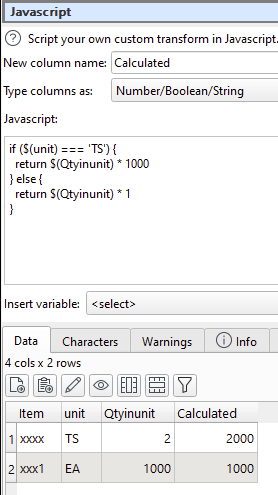# Conditional calculation

Hello everybody, I am trying to apply a calculation based on a value from a column, by example
Ap01:
Item unit Qtyinunit
xxxx TS 2
xxx1 EA 1000

The calculation that I need to do is:
if unit = “TS”
then \$(Qtyinunit)*1000
else \$(Qtyinunit)*1

problem is, that in new column it appears the calculation as string (21000) or (10001) [not evaluated]

Ap02:
I had done in another way, but takes more transformations:
(column name: Qt01)
if unit = TS
then \$(Qtyinunit)
else 0

(column name: Qt02)
if unit not equal TS
then \$(Qtyinunit)
else 0

(column name: Qt001
calculate
Qt01 * 1000

(column name: QTF)
calculate
QT001 + Qt02

If the first approach, Ap01, had worked, simplicity was welcomed (I really do not understand why expression was not evaluated…)

Miguel

Hi Miguel

The If transform doesn’t accept an expression, just a value. So you just need 2 steps to make this work.

Or you could do it in one transform using the Javascript transform.

2 Likes

Thanks, it works for me (it is shorter that second approach!),

Regards,

Miguel

1 Like

About JavaScript, I had tried to code a solution, but my knowledge in the language is limited (I used to be a Pascal coder, Basic, and FORTRAN [yes, long time ago]). Could you recommend me a good tutorial in JavaScript just to use to extend some transformations, please?

Hi,

The simplest is done by using the JavaScript transform as followsHope this helps

1 Like

This is the Javascript equivalent:

The documentation has some examples:

And there are loads of resouces online for learning Javascript. For example:JavaScript Tutorial

But I would recommend sticking with Easy Data Transform standard transforms where possible and only using JS if there is no other way to do it.

Thanks! I am eager to apply JavaScript!

Yes, I know that using JavaScript have some performance issues

The Javascript transform is still plenty fast enough for most purposes. Also if you can replace several transforms with 1 Javascript transform, then less memory is required. But I would still other ‘native’ transforms where possible.